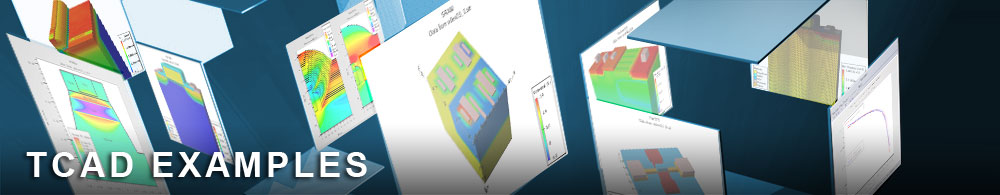NPN - AC Frequency Response

## bjtex06.in : NPN - AC Frequency Response

Requires: S-Pisces
Minimum Versions: Atlas 5.28.1.R

This example calculates the frequency response of an NPN transistor at a single DC bias point. It shows:

• Formation of an NPN BJT using Atlas syntax
• DC ramp of Vbe up to 0.8V, with Vce=2.0V
• AC simulation with ramped frequency

The device structure and DC biasing of this device are as described in the NPN Gummel plot example in this section. All models and numerical methods are in common with the other NPN examples.

The key line of syntax is:
solve prev ac freq=1e6 aname=base fstep=5 mult.f nfstep=10 .
This line specifies the solution of the previous DC bias point with a small AC signal. The freq parameter sets the initial frequency. aname sets the contact on which the AC signal is applied. If no aname is given the AC signal is applied to all contacts in turn. The fstep parameter sets the step in frequency, nfstep set the number of frequency steps and mult.f indicates that this is a multiplier. At each step the frequency is multiplied by mult.f rather than having mult.f added to the initial frequency.

The resulting capacitance and conductance parameters are stored in the log file. They can be plotted against log(frequency) to see the frequency response in TonyPlot.

To load and run this example, select the Load button in DeckBuild > Examples. This will copy the input file and any support files to your current working directory. Select the Run button in DeckBuild to execute the example.